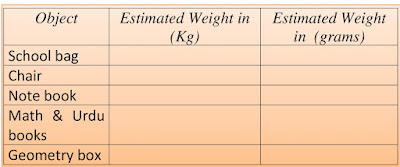# Lesson Plan of Units of Mass / Weight

Lesson Planning of Conversion of Units of Mass / Weight

Subject Mathematics

Students` Learning Outcomes

• Convert kilograms to grams and vice versa.

Information for Teachers

• Kilogram and gram are the units to measure “weight”. Kilo means thousand that is short for ‘kilogram’.
• 1 kilogram = 1,000 gram.
• A paper clip weighs about 1 gram.
• Kilogram is written as ‘kg’ and grams as ‘g’.
• A gram is about a quarter of a teaspoon of sugar, cubic centimeter of water, pen cap or a pinch of salt.• A kilogram is about 7 apples, two loaves of bread or the weight of 1 liter of water.
• To convert kilograms into grams we multiply, as; g = kg x 1,000
• To convert grams into kilograms we divide, as; kg = g ÷ 1000• During this lesson the teacher should also consult with textbook at each level wherein and whenever it is required.

Material / Resources

Writing board, chalk/marker, duster, measuring tape, scale, and textbook

Introduction

• Tell them that today we will study about converting the kg into g.
• Ask them that we measure the weight of whom, why and how?
• Ask from students do they know how much is their weight and they measure the weight with which unit?
• Tell them that our weight can be measure in kilograms.
• Ask them, “Have they even seen a balance?”
• Show them a bathroom scale and measure their weight on it.

Development

 Activity 1 Conversion of kilograms into grams: Select a fat student from the class and weigh him in kilograms in front of the class. Write his weight on the board e.g. 30 kg and tell them, to convert kilograms to grams  we multiply grams with 1000, as; Weight of the student = 30 kg Grams = kilograms x 1000 = 30 x 1000 = 30000 g Conversion of grams into kilograms: Ask a student to give his school bag and weigh it in grams in front of the class. Write on the board its weight in grams e.g. 4000 g and tell the students to convert grams into kilograms we divide grams with 1000. Weight of the bag = 4000 g Kilograms = g ÷ 1000 = 4000 / 1000 = 4 kg.Activity 2 Make the following table on the board. Ask the students to estimate the weight of given objects in kilograms and convert into grams as well.Activity 3 Explain them if the weight is given in both measures i.e. “kg and g” then first convert kilograms into grams and then add the grams into grams, as; 11 kg, 5 g = (11 x 1000 + 5) g = 11005 g. Give such sort of more examples to the students so they can fully understand the concept.

Sum up / Conclusion

• Finish the lecture by repeating some key points of the lecture.
• Kilograms and gram are the units to measure “weight”.
• Kilograms are used to measure heavy weight objects and grams to measure light weight objects.
• Kilo means thousand and 1 kilogram = 1,000 grams.
• Kilo-gram is generally written as kg and grams as g.
• To convert kilograms into grams we multiply.
• To convert grams into kilograms we divide.
• If the weight is given in both measure e.g. “kg and g” then first convert kilograms into grams and then add the grams in grams.

Assessment

• Assess the students by asking them to convert these weights into g: as;
• i)                    2.6 kg        = ________ g.
• ii)                  5 kg, 9 g     = ________ g.
• iii)                21 kg           = ________g.
• iv)                8 kg, 260 g  = ________ g.